# Neural style transfer —— Class review

Last updated on：a month ago

Neural style transfer is different from many of the algorithms you’ve seen up to now, because it doesn’t learn any parameter, instead it learns directly the pixels of an image.

# Deep ConvNets Learning

Visualizing what a deep network is learning
Pick a unit in layer 1. Find the nine image patches that maximize the unit’s activation.

A “content” image ( c) and a “style” image (S) , to create a “generated” image (G)

## Cost function

$$J(G) = J_{content}(C, G) + \beta J_{style} (S, G)$$

Find the generated image $G$
1.Initiate G randomly
G: $100 \times 100 \times 3$
2.Use gradient descent to minimize $J(G)$
$$G:= G - \frac{\alpha}{\alpha G} J(G)$$

## Content cost function

$$J(G) = \alpha J_{content} (C, G) + \beta J_{style} (S, G)$$

• Say you use hidden layer $l$ to compute content cost.

$l$ is chosen in the middle of neural network, neither too shallow nor too deep

• Use pre-trained ConvNet
• Let $a^{[l] ( c)}$ and $a^{[l] (G)}$ be the activation of layer $l$ on the images
• If $a^{[l] ( c)}$ and $a^{[l] (G)}$ is similar, both images have similar content

$$J_{content} (C, G) = 1/2 \Vert a^{[l] ( c )} - a^{[l] (G)}\Vert^2$$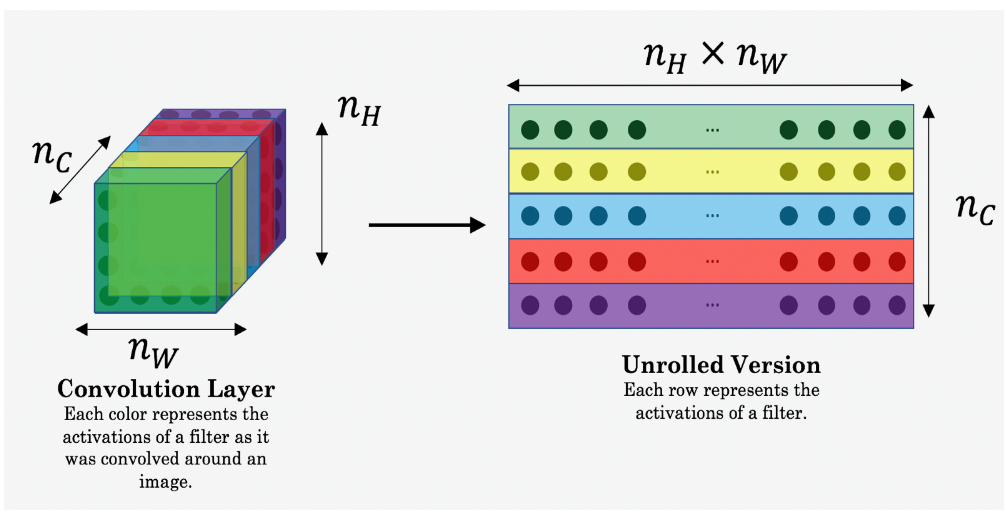$$J_{content} (C,G) = \frac{1}{4 \times n_H \times n_W \times n_C} \sum_{ \text{all entries}} (a^{( C )} - a^{( G )} )^2$$

## Style cost function

Meaning of the “style” of an image, say you are using layer $l$'s activation to measure “style”
Correlation between activations across channels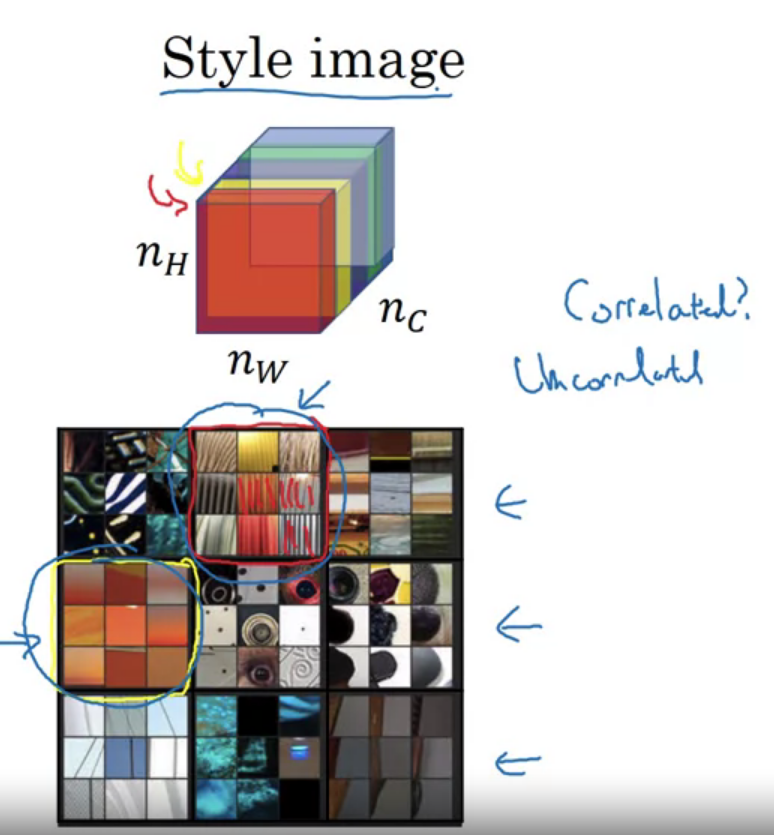$$J_{\text{style}}^{[l]}(S,G) = \frac{1}{4 \times {n_C}^2 \times (n_H \times n_W)^2} \sum_{i=1}^{n_C} \sum_{j=1}^{n_C} (G^{(S)}_{ (gram) i,j} - G^{(G)}_{ (gram) i,j})^2$$

It has order:
tf.transpose(tf.reshape($a_S, [n_H * n_W,n_C]$)) $\neq$ tf.reshape($a_S, [ n_C, n_H * n_W]$)
The dimension of latter one is right, but it is forced to be in this dimension. And the right values’ order is not we want to get.

## What you should remember

• The content cost takes a hidden layer activation of the neural network, and measures how different $a^{( c)}$ and $a^{(G)}$ are.
• When you minimize the content cost later, this will help make sure $G$ has similar content as $C$.

# Style matrix

• In linear algebra, the Gram matrix G of a set of vectors $(v_{1},\dots ,v_{n})$ is the matrix of dot products, whose entries are $G_{ij} = v_{i}^T v_{j} = \text{np.dot}(v_{i}, v_{j})$.
• In other words, $G_{ij}$ compares how similar $v_i$ is to $v_j$: If they are highly similar, you would expect them to have a large dot product, and thus for $G_{ij}$ to be large.

Let $a^{[l]}_{i, j, k}$ = activation at $(i, j, k)$.
$$G^{[l]} is n_c^{[l]} \times n_c^{[l]}$$

$$G^{[l] (S)}_{kk^{‘}} = \sum^{ n_H^{[l]} }_{ i = 1} \sum^{ n_W^{[l]} }_{ j = 1} a^{[l] (S)}_{ijk} a^{[l] (S)}_{ijk^{’}}$$

$$G^{[l] (G)}_{kk^{‘}} = \sum^{ n_H^{[l]} }_{i = 1} \sum^{ n_W^{[l]} }_{j = 1} a^{[l] (G)}_{ijk} a^{[l] (G)}_{ijk^{’}}$$

“gram matrix”

If you want the generated image to softly follow the style image, try choosing larger weights for deeper layers and smaller weights for the first layers. In contrast, if you want the generated image to strongly follow the style image, try choosing smaller weights for deeper layers and larger weights for the first layers.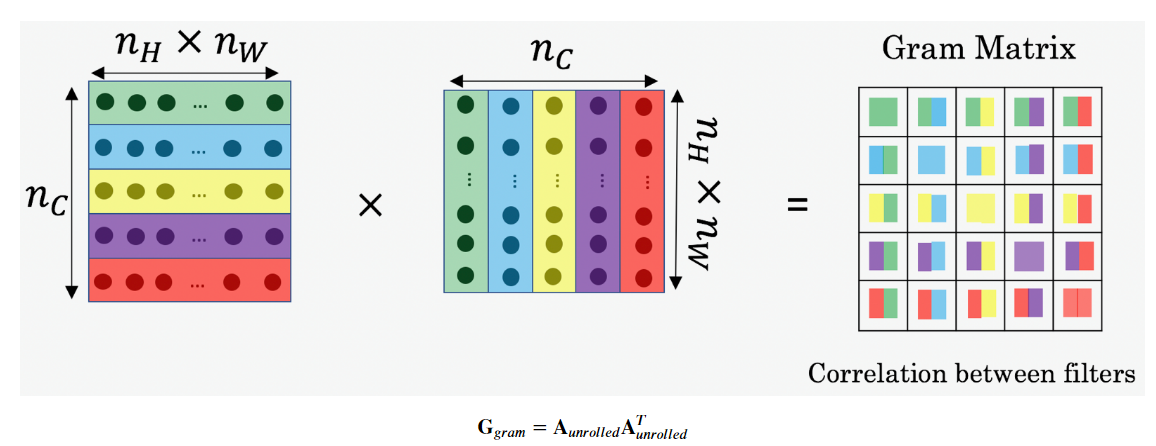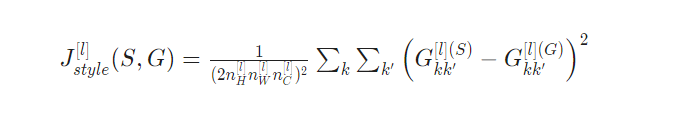• The style of an image can be represented using the Gram matrix of a hidden layer’s activations.
• You get even better results by combining this representation from multiple different layers.

Define the total cost to optimize

$$J(G) = \alpha J_{content}(C,G) + \beta J_{style}(S,G)$$

# 1D and 3D generalizations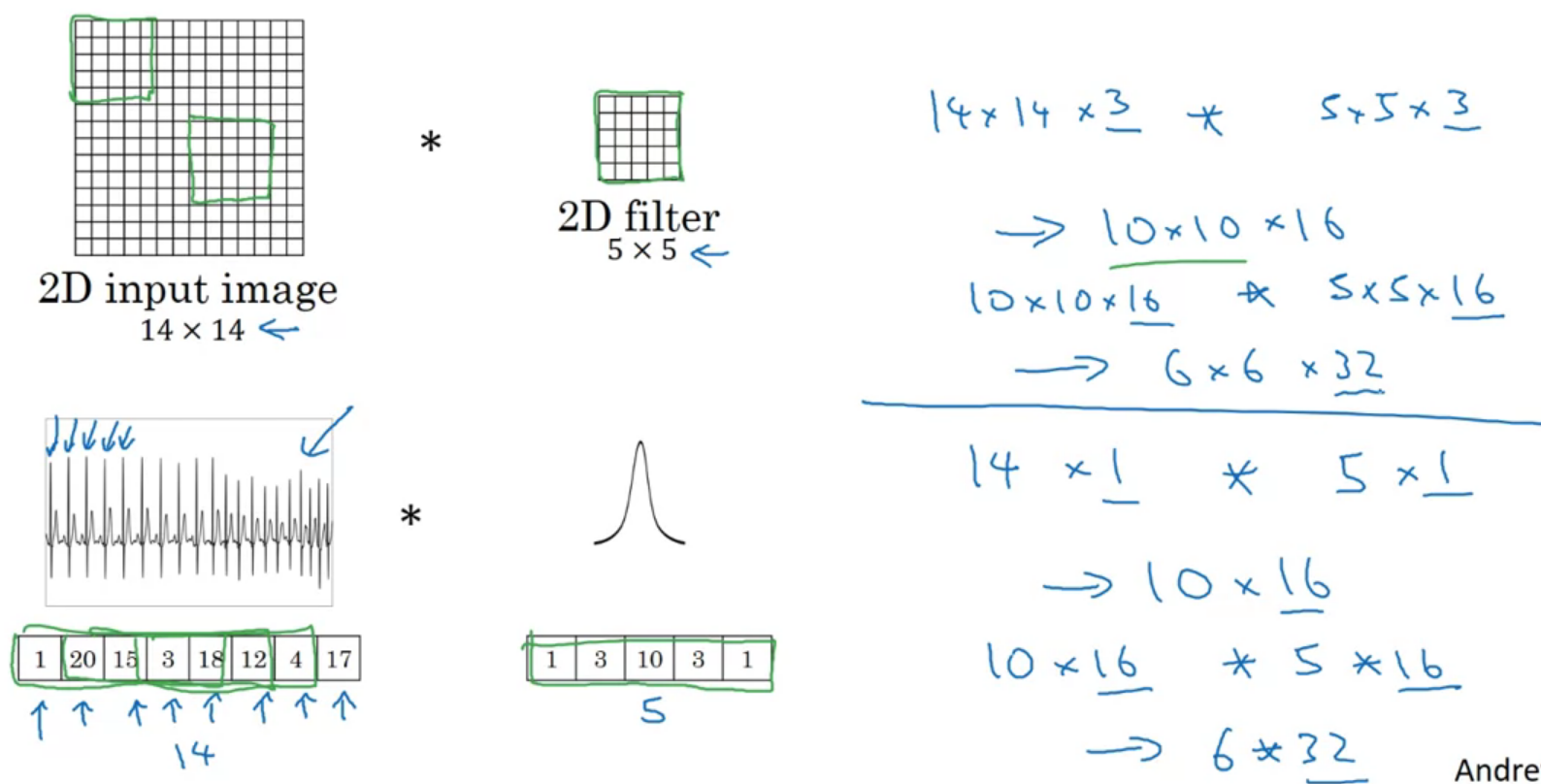3D filter detects features across your 3D data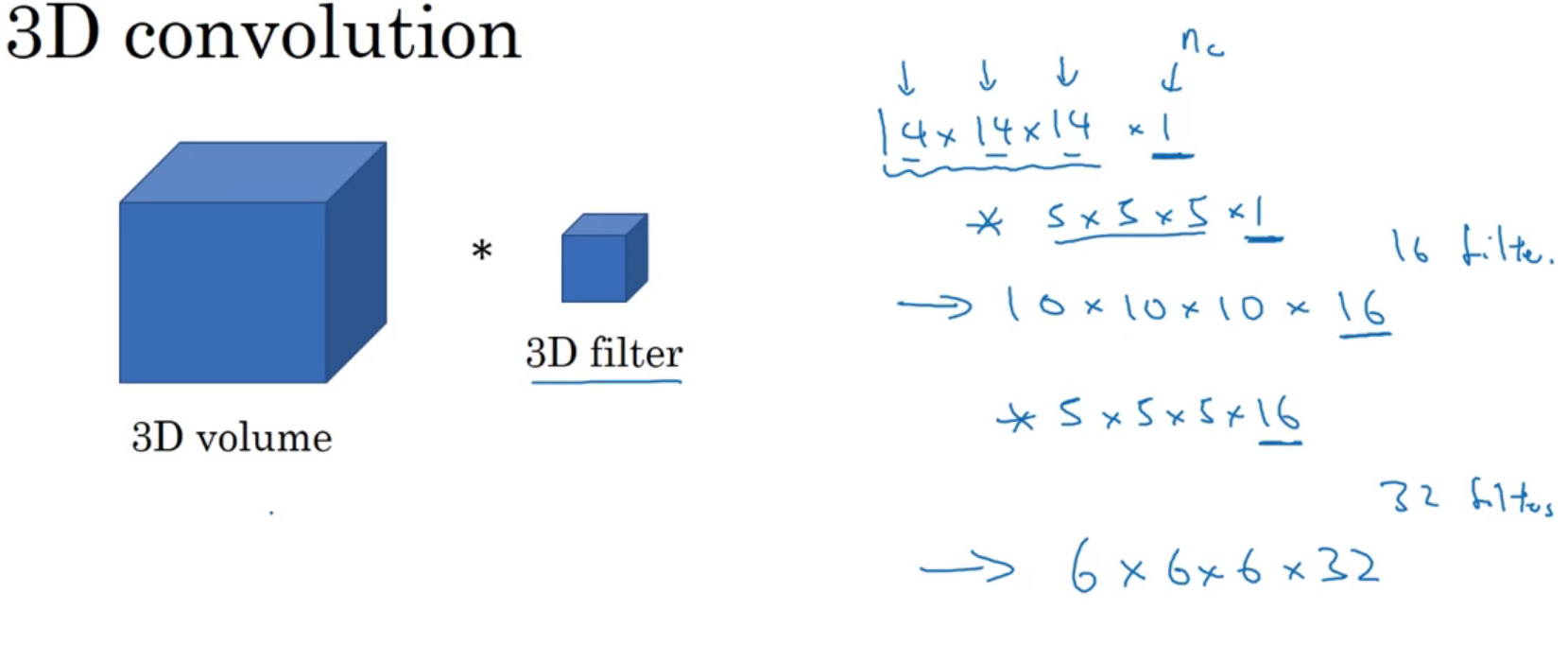# What you should remember

• Neural Style Transfer is an algorithm that given a content image C and a style image S can generate an artistic image
• It uses representations (hidden layer activations) based on a pretrained ConvNet.
• The content cost function is computed using one hidden layer’s activations.
• The style cost function for one layer is computed using the Gram matrix of that layer’s activations. The overall style cost function is obtained using several hidden layers.
• Optimizing the total cost function results in synthesizing new images.

# Q&A

In neural style transfer, what is updated in each iteration of the optimization algorithm?

The pixel values of the generated image $G$.

6.You train a ConvNet on a dataset with 100 different classes. You wonder if you can find a hidden unit which responds strongly to pictures of cats. (I.e., a neuron so that, of all the input/training images that strongly activate that neuron, the majority are cat pictures.) You are more likely to find this unit in layer 4 of the network than in layer 1

this neuron understands complex shapes (cat pictures) so it is more likely to be in a deeper layer than in the first layer.

7.Neural style transfer is trained as a supervised learning task in which the goal is to input two images (xx), and train a network to output a new, synthesized image (yy).

False: Neural style transfer is about training on the pixels of an image to make it look artistic, it is not learning any parameters.

# Reference

 Deeplearning.ai, Convolutional Neural Networks